# Fubini-Study metric

(diff) ← Older revision | Latest revision (diff) | Newer revision → (diff)

The Hermitian metric on a complex projective space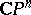defined by the Hermitian scalar product in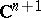. It was introduced almost simultaneously by G. Fubini  and E. Study . The Fubini–Study metric is given by the formulawhereis the scalar product inand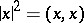; the distance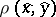between the points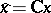,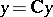, where, is determined from the formula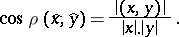The Fubini–Study metric is Kählerian (and is even a Hodge metric); its associated Kähler form is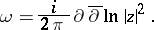The Fubini–Study metric is, up to proportionality, the unique Riemannian metric onthat is invariant under the unitary group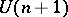, which preserves the scalar product. The space, endowed with the Fubini–Study metric, is a compact Hermitian symmetric space of rank 1. It is also called an elliptic Hermitian space.